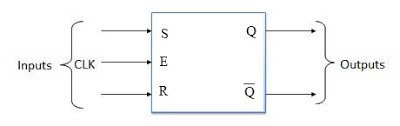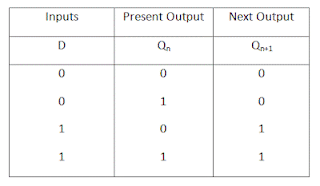# Flip-Flops | Memory Element | Types (SR, JK, T, D-type)

A bistable memory elements used in sequential circuits to store binary information are called flip-flops. A flip-flop is a binary cell capable of...

## Flip-flops (Memory Element)

A bistable memory elements used in sequential circuits to store binary information are called flip-flops. A flip-flop is a binary cell capable of storing one bit of information. It has two outputs, one for the normal value and one for the compliment value of the bit stored in it. The circuit can be made to change state by signals applied to one or more control inputs and will have one or two outputs. It is the basic storage element in sequential logic.

Flip-flops and latches are fundamental building blocks of digital electronics systems used in computers, communications and many other types of systems.

## Types of flip-flop

There are several different kinds of flip-flop circuit, with designation such as S-R (set/reset), J-K (Jack Kilby), D (delay) and T (toggle). A flip-flop typically includes zero, one, or two input signals as well as a clock signal and output signal. The most common types of flip-flops are presented below in Fig.

### (i) SR Flip-flop

The graphic symbol of SR flip-flop is shown in Fig. It has three inputs, labeled S (for set), R (for reset), and C (for clock). It has an output Q and sometimes the flip-flop has a complemented output, which is indicated with a small circle at the other output terminal. There is an arrowhead-shaped symbol in front of the letter C to designate a dynamic input.Blok Diagram of SR flip-FlopCircuit DiagramTruth table

Operation:- The operation of the SR flip-flop is as follows. If there is no signal at the clock input C, the output of the circuit cannot change irrespective of the values at inputs S and R. Only when the clock signal changes from 0 to 1 can the output be affected according to the values in inputs S and R. If S=1 and R=0 when C changes from 0 to 1 output Q is set to 1. If both S and R are 0 during the clock transition, the output does not change. When both S and R are equal to 1, the output is unpredictable and may go to either 0 or 1, depending on internal timing delays that occur within the circuit.

### (ii) JK flip-flop

A JK flip-flop is a refinement of the SR flip-flop in that the indeterminate condition of the SR type is defined in the JK type. Inputs J and K behave like inputs S and R to set and clear the flip-flop, respectively. When inputs J and K are both equal to 1, a clock transition switches the outputs of the flip-flop to their compliment state.

The graphic symbol and characteristic table of the JK flip-flop are shown in Fig. The J input is equivalent to the S (set), and K input is equivalent to the R (reset) input of the SR flip-flop. Instead of the indeterminate condition, the JK flip-flop has a complement condition Q(t+1) = Q'(t) when both J and K are equal to 1.

### (iii) D Flip-flop

The D (delay) flip-flop is a slight modification of the SR flip-flop. An SR flip-flop is converted to a D flip-flop by inserting an inverter between S and R and assigning the symbol D to the single input. If D=1 the output of the flip-flop goes to the 1 state, but if D=0, the output of the flip-flop goes to the 0 state.

The graphic symbol and characteristic table of the D flip-flop are shown in Fig. From the characteristic table we note that the next state Q(t+1) is determined from the D input. The relationship can be expressed by a characteristic equation:

Q(t+1) = D

This means that the Q output of the flip-flop receives its value from the D input every time that the clock signal goes through a transition from 0 to 1.Logic diagram D flip-flopTruth table of D flip-flop

### (iv) T Flip-flop

The T (toggle) flip-flop is obtained from a JK type when inputs J and K are connected to provide a single input designated by T. The T flip-flop therefore has only two conditions. When T=0 (J=K=0) a clock transition does not change the state of the flip-flop. When T=1 (J=K=1) a clock transition compliments the state of the flip-flop. These conditions can be expressed by a characteristic equation:

Q(t+1) = Q(t)+T

## Application

Flip-flops and latches are used as data storage elements. It can also be used for counting of pulses and for synchronizing variable-timed input signals to same reference timing signal.